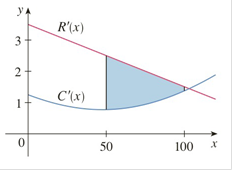Chapter 5.1, Problem 54E

Chapter
Section
Textbook Problem

# The figure shows graphs of the marginal revenue function R′ and the marginal cost function C′ for a manufacturer. [Recallfrom Section 3.7 that R ( x ) and C(x) represent the revenue and cost when x units are manufactured. Assume that R and C are measured in thousands of dollars.] What is the meaning of the area of the shaded region? Use the Midpoint Rule to estimate the value of this quantity.To determine

To explain:

The meaning of the area of the shaded region and use midpoint rule to estimate the value of the quantity

Explanation

1) Concept:

Midpoint rule baf(x)dxi=1nfxi-x=x[fx1-++fxn-]

where, x=b-an and

xi-=12xi-1+xi= Midpoint of [xi-1, xi]

2) Calculation:

The area under R'(x) from x=50 to x=100 represents the change in revenue, and the area under C'(x) represents the change in cost.

So the shaded region represents the difference between the areas under R'xand C'(x)

Let P'x=R'x- C'(x)

The rate of increase in the profit as production levels change from 50 to 100 units is R'x- C'(x). This is marginal profit function. So the shaded area represents total profit as production levels change from 50 to 100 units.

With n=5,a=50, and  b=100,the interval width is

x=b-an

Substitute values.

x=100-505

Simplify.

x=505

Simplify.

x=10

Use midpoint rule with x=10 and n=5

### Still sussing out bartleby?

Check out a sample textbook solution.

See a sample solution

#### The Solution to Your Study Problems

Bartleby provides explanations to thousands of textbook problems written by our experts, many with advanced degrees!

Get Started

#### Find more solutions based on key concepts